# Set Associative Mapping | Practice Problems

## Set Associative Mapping-

Before you go through this article, make sure that you have gone through the previous article on Set Associative Mapping.

In set associative mapping,

• A particular block of main memory can be mapped to one particular cache set only.
• Block ‘j’ of main memory will map to set number (j mod number of sets in cache) of the cache.
• A replacement algorithm is needed if the cache is full.

In this article, we will discuss practice problems based on set associative mapping.

## Problem-01:

Consider a 2-way set associative mapped cache of size 16 KB with block size 256 bytes. The size of main memory is 128 KB. Find-

1. Number of bits in tag
2. Tag directory size

## Solution-

Given-

• Set size = 2
• Cache memory size = 16 KB
• Block size = Frame size = Line size = 256 bytes
• Main memory size = 128 KB

We consider that the memory is byte addressable.

### Number of Bits in Physical Address-

We have,

Size of main memory

= 128 KB

= 217 bytes

Thus, Number of bits in physical address = 17 bits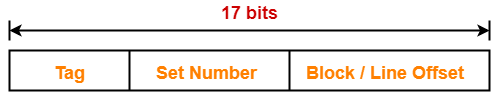### Number of Bits in Block Offset-

We have,

Block size

= 256 bytes

= 28 bytes

Thus, Number of bits in block offset = 8 bits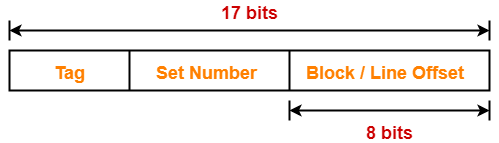### Number of Lines in Cache-

Total number of lines in cache

= Cache size / Line size

= 16 KB / 256 bytes

= 214 bytes / 28 bytes

= 64 lines

Thus, Number of lines in cache = 64 lines

### Number of Sets in Cache-

Total number of sets in cache

= Total number of lines in cache / Set size

= 64 / 2

= 32 sets

= 25 sets

Thus, Number of bits in set number = 5 bits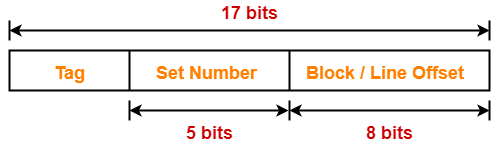### Number of Bits in Tag-

Number of bits in tag

= Number of bits in physical address – (Number of bits in set number + Number of bits in block offset)

= 17 bits – (5 bits + 8 bits)

= 17 bits – 13 bits

= 4 bits

Thus, Number of bits in tag = 4 bits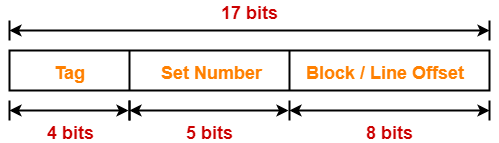### Tag Directory Size-

Tag directory size

= Number of tags x Tag size

= Number of lines in cache x Number of bits in tag

= 64 x 4 bits

= 256 bits

= 32 bytes

Thus, size of tag directory = 32 bytes

Also Read- Practice Problems On Direct Mapping

## Problem-02:

Consider a 8-way set associative mapped cache of size 512 KB with block size 1 KB. There are 7 bits in the tag. Find-

1. Size of main memory
2. Tag directory size

## Solution-

Given-

• Set size = 8
• Cache memory size = 512 KB
• Block size = Frame size = Line size = 1 KB
• Number of bits in tag = 7 bits

We consider that the memory is byte addressable.

### Number of Bits in Block Offset-

We have,

Block size

= 1 KB

= 210 bytes

Thus, Number of bits in block offset = 10 bits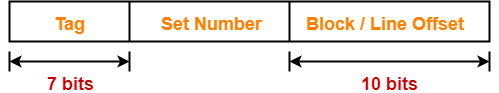### Number of Lines in Cache-

Total number of lines in cache

= Cache size / Line size

= 512 KB / 1 KB

= 512 lines

Thus, Number of lines in cache = 512 lines

### Number of Sets in Cache-

Total number of sets in cache

= Total number of lines in cache / Set size

= 512 / 8

= 64 sets

= 26 sets

Thus, Number of bits in set number = 6 bits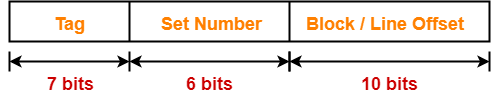### Number of Bits in Physical Address-

Number of bits in physical address

= Number of bits in tag + Number of bits in set number + Number of bits in block offset

= 7 bits + 6 bits + 10 bits

= 23 bits

Thus, Number of bits in physical address = 23 bits

### Size of Main Memory-

We have,

Number of bits in physical address = 23 bits

Thus, Size of main memory

= 223 bytes

= 8 MB

### Tag Directory Size-

Tag directory size

= Number of tags x Tag size

= Number of lines in cache x Number of bits in tag

= 512 x 7 bits

= 3584 bits

= 448 bytes

Thus, size of tag directory = 448 bytes

## Problem-03:

Consider a 4-way set associative mapped cache with block size 4 KB. The size of main memory is 16 GB and there are 10 bits in the tag. Find-

1. Size of cache memory
2. Tag directory size

## Solution-

Given-

• Set size = 4
• Block size = Frame size = Line size = 4 KB
• Main memory size = 16 GB
• Number of bits in tag = 10 bits

We consider that the memory is byte addressable.

### Number of Bits in Physical Address-

We have,

Size of main memory

= 16 GB

= 234 bytes

Thus, Number of bits in physical address = 34 bits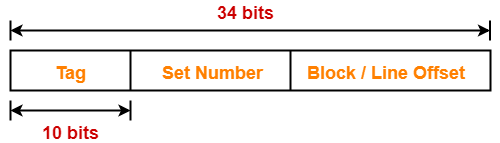### Number of Bits in Block Offset-

We have,

Block size

= 4 KB

= 212 bytes

Thus, Number of bits in block offset = 12 bits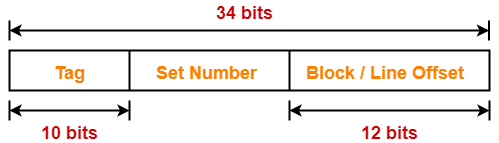### Number of Bits in Set Number-

Number of bits in set number

= Number of bits in physical address – (Number of bits in tag + Number of bits in block offset)

= 34 bits – (10 bits + 12 bits)

= 34 bits – 22 bits

= 12 bits

Thus, Number of bits in set number = 12 bits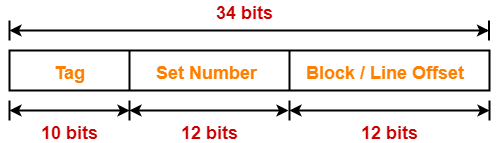### Number of Sets in Cache-

We have-

Number of bits in set number = 12 bits

Thus, Total number of sets in cache = 212 sets

### Number of Lines in Cache-

We have-

Total number of sets in cache = 212 sets

Each set contains 4 lines

Thus,

Total number of lines in cache

= Total number of sets in cache x Number of lines in each set

= 212 x 4 lines

= 214 lines

### Size of Cache Memory-

Size of cache memory

= Total number of lines in cache x Line size

= 214 x 4 KB

= 216 KB

= 64 MB

Thus, Size of cache memory = 64 MB

### Tag Directory Size-

Tag directory size

= Number of tags x Tag size

= Number of lines in cache x Number of bits in tag

= 214 x 10 bits

= 163840 bits

= 20480 bytes

= 20 KB

Thus, size of tag directory = 20 KB

## Problem-04:

Consider a 8-way set associative mapped cache. The size of cache memory is 512 KB and there are 10 bits in the tag. Find the size of main memory.

## Solution-

Given-

• Set size = 8
• Cache memory size = 512 KB
• Number of bits in tag = 10 bits

We consider that the memory is byte addressable.

Let-

• Number of bits in set number field = x bits
• Number of bits in block offset field = y bits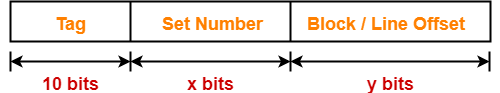### Sum of Number Of Bits Of Set Number Field And Block Offset Field-

We have,

Cache memory size = Number of sets in cache x Number of lines in one set x Line size

Now, substituting the values, we get-

512 KB = 2x x 8 x 2y bytes

219 bytes = 23+x+y bytes

19 = 3 +x + y

x + y = 19 – 3

x + y = 16

### Number of Bits in Physical Address-

Number of bits in physical address

= Number of bits in tag + Number of bits in set number + Number of bits in block offset

= 10 bits + x bits + y bits

= 10 bits + (x + y) bits

= 10 bits + 16 bits

= 26 bits

Thus, Number of bits in physical address = 26 bits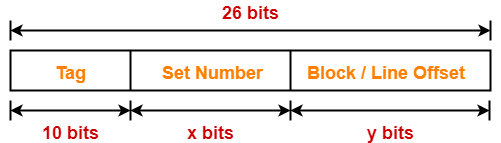### Size of Main Memory-

We have,

Number of bits in physical address = 26 bits

Thus, Size of main memory

= 226 bytes

= 64 MB

Thus, size of main memory = 64 MB

## Problem-05:

Consider a 4-way set associative mapped cache. The size of main memory is 64 MB and there are 10 bits in the tag. Find the size of cache memory.

## Solution-

Given-

• Set size = 4
• Main memory size = 64 MB
• Number of bits in tag = 10 bits

We consider that the memory is byte addressable.

### Number of Bits in Physical Address-

We have,

Size of main memory

= 64 MB

= 226 bytes

Thus, Number of bits in physical address = 26 bits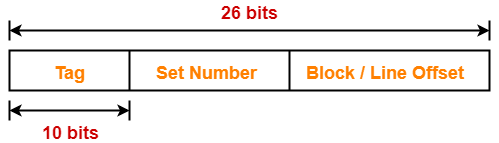### Sum Of Number Of Bits Of Set Number Field And Block Offset Field-

Let-

• Number of bits in set number field = x bits
• Number of bits in block offset field = y bitsThen, Number of bits in physical address

= Number of bits in tag + Number of bits in set number + Number of bits in block offset

So, we have-

26 bits = 10 bits + x bits + y bits

26 = 10 + (x + y)

x + y = 26 – 10

x + y = 16

Thus, Sum of number of bits of set number field and block offset field = 16 bits

### Size of Cache Memory-

Cache memory size

= Number of sets in cache x Number of lines in one set x Line size

= 2x x 4 x 2y bytes

= 22+x+y bytes

= 22+16 bytes

= 218 bytes

= 256 KB

Thus, size of cache memory = 256 KB

To watch video solutions and practice more problems,

Watch this Video Lecture

Get more notes and other study material of Computer Organization and Architecture.

Watch video lectures by visiting our YouTube channel LearnVidFun.

Summary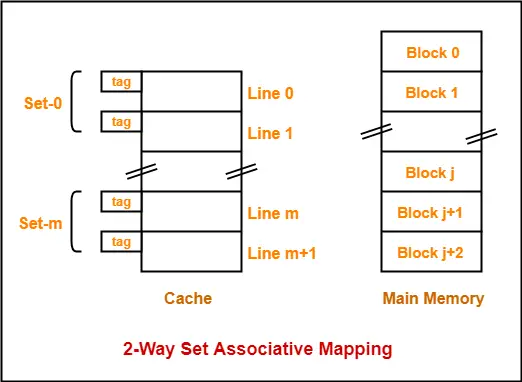Article Name
Set Associative Mapping | Practice Problems
Description
Practice Problems based on set associative mapping. Set associative mapping is a cache mapping technique that allows to map a particular block of main memory to one particular cache set only.
Author
Publisher Name
Gate Vidyalay
Publisher Logo